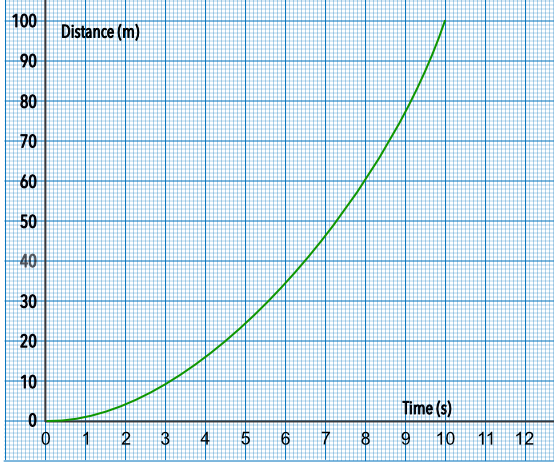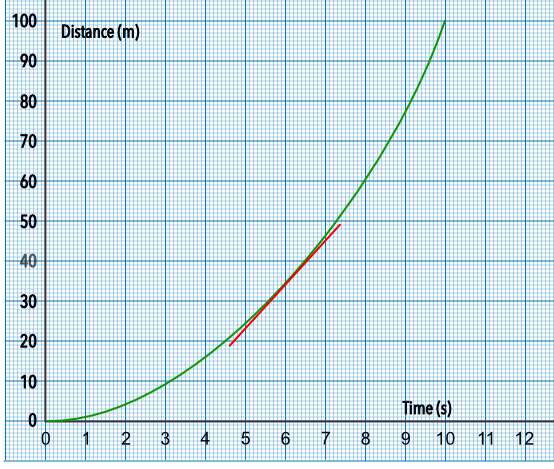Rates of Change in Context

## Rates of Change in Context

Rates of Change may be determined graphically, using a tangent to a graph; or numerically by using data to determine the gradient.

Drawing a tangent to a graph will give an instantaneous rate of change whereas using data for two points (which would be the same as drawing a chord on the graph) will give an average rate of change.

## Example 1

A racing car accelerates from stationary and covers 260m in the first six seconds. In the following six seconds, it covers a further 460m. What is the average velocity of the car over the first 12 seconds?

Total distance covered is 260 + 460 = 720m

Total time = 12 seconds

Average velocity = frac(text(distance))(text(time)) = 720 ÷ 12 = 60m/s

## Example 2

The graph shows distance against time for a train pulling away from a station. What is the speed of the locomotive at 6 seconds?Draw a tangent to the graph at 6 seconds.The gradient of the tangent gives the velocity.

gradient = frac(text(up))(text(along)) = frac(45 - 23)(7 - 5) = frac(22)(2) = 11 m/s